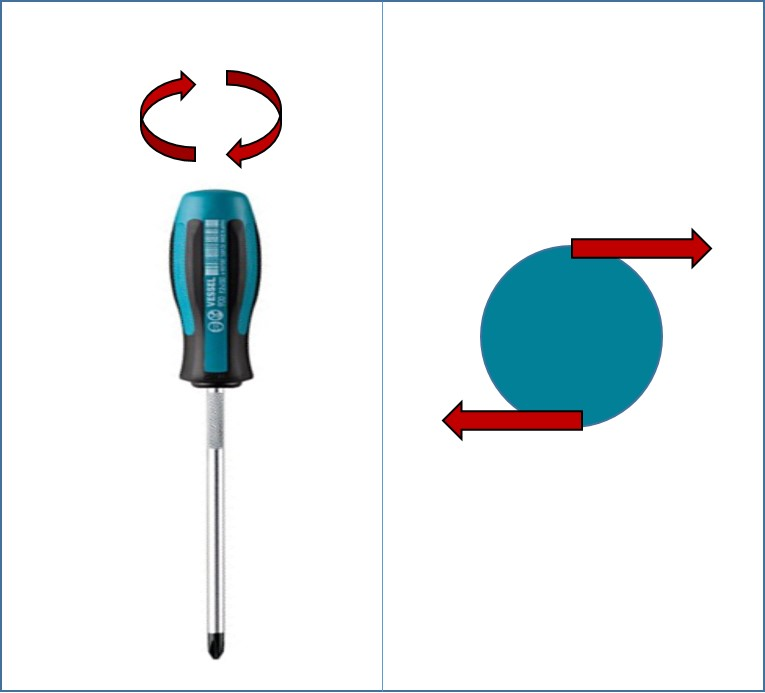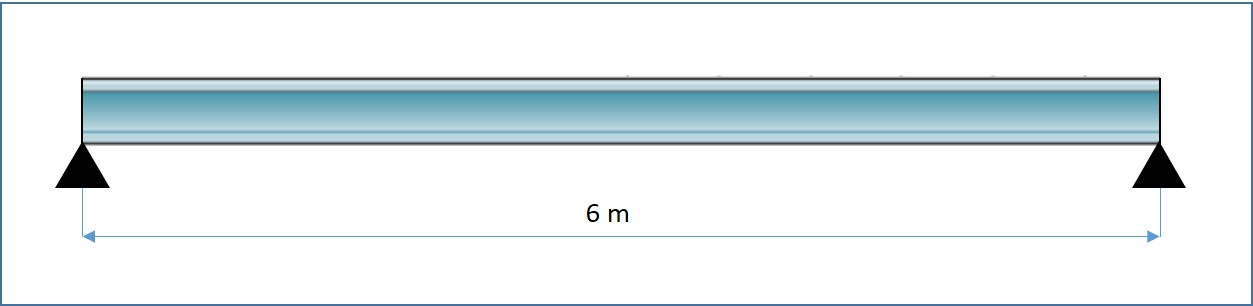# Moments

## Introduction

When a force acts on an object, the force may cause an object to move in a straight line. It could also cause the object to spin or turn. This turning effect of a force is called the moment of the force. It is defined as the product of the force and the perpendicular distance of the line of action of the force from the pivot. Since the force is measured in Newton and distance is measured in meter, the unit of the torque is Newton-meter (N m).

The following example shows moments created at the bolt head.Figure 1: Moment of a Force

A. Couple

When we tighten a screw, we apply a turning effect to the screwdriver. If we apply one force to the screwdriver, then the screwdriver would move sideways. Therefore, we apply two forces of equal size but opposite direction on opposite sides of the screwdriver handle. This set of two equal forces in opposite direction is called a couple.Figure 2: Couple

B. Torque

Consider two parallel forces, each of magnitude (F) acting on a bolt head, each force produces a moment about the centre of the bolt head. The total moment about the centre is the product of force and the perpendicular distance between the forces. When a turning effect is produced by two forces, the resulting turning effect is called torque.C. Principle of Moments

The principle of moments states that for a body to be in rotational equilibrium, the sum of the clockwise moments about any point must be equal to the sum of the anticlockwise moments about that same point.

D. Centre of Mass

An object may be made to balance at a particular point. When it is balanced at this point, the object does not turn and so all the weight on one side of the pivot is balanced by the weight on the other side. This point is called the centre of gravity of the object.

E. Practical Impact of Moments

Moment and torque are important concepts in designing automobile parts. Engine crank shafts, propeller shafts and many other rotating components are designed to withstand torque acting on them. Centre of gravity is very important in the construction industry. When operating cranes, a crane operator should always ensure that centre of gravity is maintained within the crane body to avoid crane overturn.Figure 3: Centre of Gravity

F. Example Application

A uniform beam, as shown in Figure 4, is horizontal and it is supported at two points. The mass of the beam is 2500 N. Calculate the moment acting on each support.Figure 4: Uniform Beam Supported at Two Points

Solution:

A vector diagram for the above example is given in Figure 5. The total mass of the beam is represented by force (F), acting at the centre of the beam. The perpendicular distance between the pivot point and the force is half of the beam length.Figure 5: A Vector Diagram of Figure 4

Moment of force = force x perpendicular distance from the pivot.

= 2500 x 3

= 7500 N m

G. Summary

• The moment of force is a product of the force and the perpendicular distance of the line of action of the force from the pivot.
• Two forces, equal in magnitude but opposite in direction whose lines of action do not coincide, form a couple.
• Torque is defined as a product of force and the perpendicular distance between the forces in a couple.
• For a body to be in rotational equilibrium, the sum of the clockwise moments about any point must be equal to the sum of the anticlockwise moments about that same point.